GET THE APP

Approximate Solution to First-order Integro-differential Equations Using Polynomial Collocation Approach

# Journal of Applied & Computational Mathematics

ISSN: 2168-9679

Open Access
Submit Manuscript arrow_forward arrow_forwardResearch Article - (2022) Volume 11, Issue 8

# Approximate Solution to First-order Integro-differential Equations Using Polynomial Collocation Approach

Ganiyu Ajileye* and F.A. Aminu
*Correspondence: Ganiyu Ajileye, Department of Mathematics and Statistics, Federal University Wukari, Taraba, Nigeria, Email:
Department of Mathematics and Statistics, Federal University Wukari, Taraba, Nigeria

Received: 04-Aug-2022, Manuscript No. JACM-22-73430; Editor assigned: 06-Aug-2022, Pre QC No. P-73430; Reviewed: 12-Aug-2022, QC No. Q-73430; Revised: 18-Aug-2022, Manuscript No. R-73430; Published: 24-Aug-2022 , DOI: 10.37421/2168-9679.2022.11.486
Citation: Ajileye, Ganiyu and Aminu F.A. “Approximate Solution to First-Order Integro-differential Equations Using Polynomial Collocation Approach.” J Appl Computat Math 11 (2022): 486.
Copyright: © 2022 Ajileye G, et al. This is an open-access article distributed under the terms of the Creative Commons Attribution License, which permits unrestricted use, distribution, and reproduction in any medium, provided the original author and source are credited.

## Abstract

In this study, power series and shifted Chebyshev polynomials are used as basis function for solving first order volterra integro-differential equations using standard collocation method. An assumed approximate solution in terms of the constructed polynomial was substituted into the class of integro-differential equation considered. The resulted equation was collocated at appropriate points within the interval of consideration [0,1] to obtain a system of algebraic linear equations. Solving the system of equations, by inverse multiplication, the unknown coefficients involved in the equations are obtained. The required approximate results are obtained when the values of the constant coefficients are substituted back into the assumed approximate solution. Numerical example are presented to confirm the accuracy and efficiency of the method.

#### Keywords

Integro-differential equations • Collocation • Power series polynomial • Shifted chebyshev polynomial

#### Introduction

An Integro-differential equation is any type of equation combining an unknown function involving both integral and derivative operators. It is applicable in several fields, such as engineering and science. These equations are either Fredholm, Volterra, or Fredholm-Volterra integro-differential equations. As a result, a numerical method for solving such equations is required. Some numerical approaches in literature for solving integro-differential equations include: Collocation method [1-5], Hybrid linear multistep method [6-8], Differential transform method , Perturbed Method , Homotopy Perturbation , Bernoulli matrix method , The Mellin transform approach  and Pseudospectral Method . Shahooth et al. presented a numerical method for solving the linear Volterra-Fredholm integro-differential equations of the second kind . This method is called the Bernestein polynomial method. This technique transforms the integro-differential equations into the system of algebraic equations. Some numerical results are presented to illustrate the efficiency and accuracy of this method.

In this work, we consider Volterra Integro-differential equation of the form: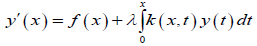Subject to initial condition;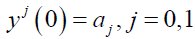Where yj = djy / dyj y(x) is the unknown function, f(x) is a known function, λ is a known constant and k(x,t) is the integral kernel function.

Basic Definitions

This section defines the basic terms that will be frequently used during the research work.

Definition 1: Standard Collocation Method: This is the method partitioning intervals into different points. For example, let say an interval [a,b], N be the number of collocation points to get the desired collocation points, we use;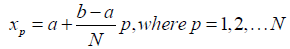Definition 2: Power Series Polynomial: A power series is basically an infinite degree polynomial that represents some function. It is generally written in the form;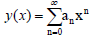Where an is the coefficient of the polynomials which are real numbers.

Definition 3: Shifted Chebyshev Polynomials: This is a special type of polynomial generated from Chebyshev polynomial. The shifted Chebyshev has interval [0,1], while Chebyshev has an interval of [-1,1]. The shifted Chebyshev is generated using;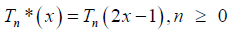where Tn (x) is the shifted chebyshev term,while Tn (x) is the chebyshev term.

Few terms of the shifted Chebyshev terms are;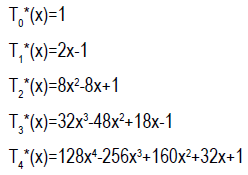#### Methodology

In this section, combination of collocation method and power series approximation is employed for the numerical solution of volterra integrodfferential equations.

Let the solution to (1) and (2) be approximated by (3)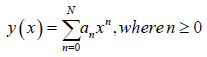Where an is the real coefficients to be determined, differenting (3) with respect to x, gives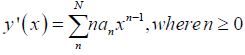substituting (3) and (4) into (1), gives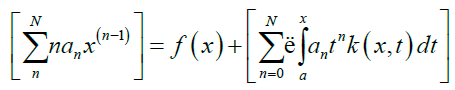simplifying (5) gives,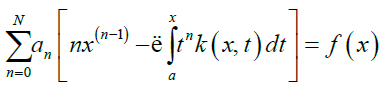Hence,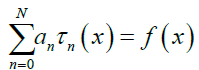Where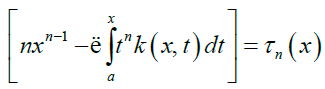Equation (6) can be written in the form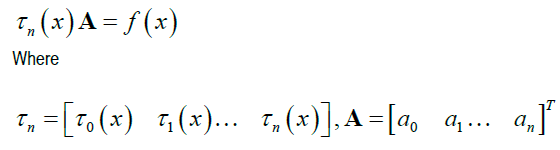Collocating equation (7) using the standard collocation points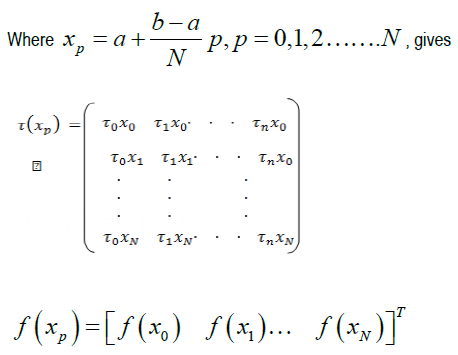Considering the initial condition,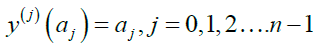Substituting (3) into (9) we have,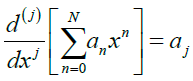Using equation(10) and equation (8) gives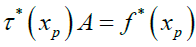Solving equation (11) using inverse multiplication to get the values of the unknown coefficient an, n=1, 2, …N and substituting the value of the coefficients into the approximate solution gives the numerical results. Repeating the above steps using shifted Chebyshev polynomial as the basis polynomial function, we obtained the approximate solution.

Numerical Examples: In this section, numerical examples with initial conditions are solved to confirm the efficiency and accuracy of the method. Let y_n (x) and y(x) be the approximate and exact solution respectively. Error_N = |y_n (x)-y(x)|.

Example 1: Considering Volterra integro-differential equation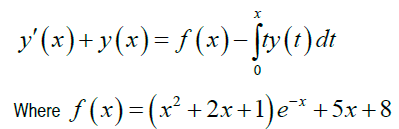subject to initial condition y(0)=10

Exact solution: y(x)=10-xe-x

Solution 1: Solving at N = 5 using power series polynomial. Substituting approximate solution (3) into example 1 gives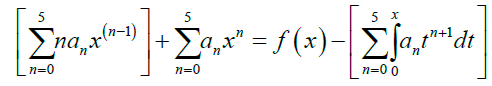Collecting the like terms gives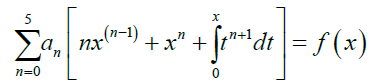Equation (12) can be written in the form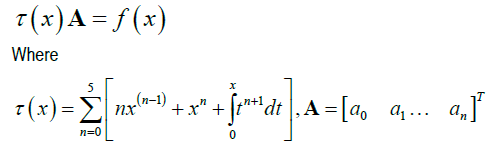collocating at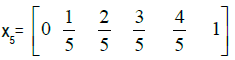and substituting the initial conditions gives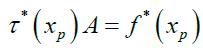Solving equation (14), we obtained the following approximate solutionUsing the above steps for solving shifted Chebyshev polynomial as the basis polynomial function, we obtained the approximate solution;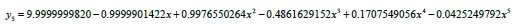Example 2: Considering Volterra integro-differential equation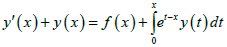Where f(x)=0

subject to initial condition y(0)=1

Exact solution: y (x) = e−xcosh (x)

Solution 2: Solving at N = 5 using power series polynomial. Substituting approximate solution (3) into example 2 gives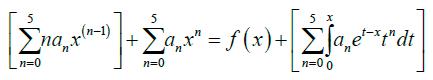Collecting the like terms gives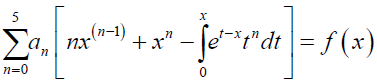Equation (15) can be written in the form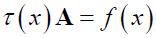Where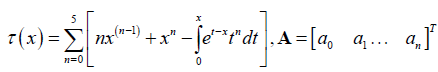collocating at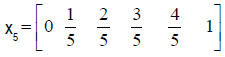and substituting the initial conditions gives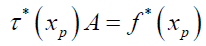Solving equation (17), we obtained the following approximate solution (Tables 1-4).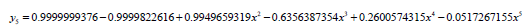Table 1: Exact and approximate values of example 1.

Xi Exact Solution Power Seriesn=5 Shifted Chebyshevn=5
0 10 9.999999994 9.999999982
0 9.909516258 9.909509989 9.909508005
0 9.836253849 9.836242518 9.836278452
0 9.777754534 9.77774555 9.777945271
0 9.731871982 9.731868389 9.732450172
1 9.69673467 9.696733792 9.69799158
1 9.670713018 9.670710096 9.67297361
1 9.652390287 9.652383354 9.655955045
1 9.640536829 9.640529449 9.645598296
1 9.634087306 9.634086223 9.640618379
1 9.632120559 9.632125613 9.639731878

Table 2: Absolute error for example 1.

Xi Power SeriesN=5 Shifted Chebyshevn=5
0 6.00E-09 1.70E-08
0 6.27E-06 8.25E-06
0 1.13E-05 2.46E-05
0 8.98E-06 1.91E-04
0 3.59E-06 5.78E-04
1 8.78E-07 1.26E-03
1 2.92E-06 2.26E-03
1 6.93E-06 3.56E-03
1 7.38E-06 5.06E-03
1 1.08E-06 6.53E-03
1 5.05E-06 7.61E-03

Table 3: Exact and approximate values of example 2.

Xi Exact Solution Power Seriesn=5 Shifted Chebyshevn=5
0 1 0.999999938 0.999999916
0.1 0.909365377 0.90934122 0.909281555
0.2 0.835160023 0.835116552 0.834695109
0.3 0.774405818 0.774370716 0.773063213
0.4 0.724664482 0.724648492 0.72176967
0.5 0.68393972 0.683932578 0.678688419
0.6 0.650597106 0.650581523 0.642112506
0.7 0.623298482 0.623267655 0.610683052
0.8 0.600948259 0.600915006 0.583318222
0.9 0.582649444 0.582637242 0.559142197
1 0.567667642 0.567675589 0.537414142

Table 4: Absolute error of example 2.

Xi Power SeriesN=5 Shifted ChebyshevN=5
0 6.24E-08 8.43E-08
0.1 2.42E-05 8.38E-05
0.2 4.35E-05 4.65E-04
0.3 3.51E-05 1.34E-03
0.4 1.60E-05 2.89E-03
0.5 7.14E-06 5.25E-03
0.6 1.56E-05 8.48E-03
0.7 3.08E-05 1.26E-02
0.8 3.33E-05 1.76E-02
0.9 1.22E-05 2.35E-02
1 7.95E-06 3.03E-02

#### Conclusion

The numerical solution of the Volterra integro-differential equation is presented in this study and the results are compared to the exact solution. Power series and shifted Chebyshev polynomials are used as the basis functions.

The numerical results of the examples as shown in tables shows that the results of power series approximation converges to the exact solution than the results of shifted chebyshev approximation. Observation also shows that as the value of x increases i.e. (from 0 to 1), the results of the shifted chebyshev diverges from the exact solutions.

None.

#### References

1. Olumuyiwa, A. Agbolade and Timothy A. Anak. "Solution of first order Volterra linear integro-differential equations by collocation method." J Appl Math 1510267 (2017).
2. Adesanya, A. O., Yahaya Y. A., Ahmed B. and Onsachi R. O. "Numerical Solution of Linear Integral and Integro-Differential Equations Using Boubakar Collocation Method." Intern J Math Sci Optim Theory Appl 2019 (2019): 592-598.

4. Gegele, O. A., Evans O. P. and Akoh D. "Numerical Solution of Higher Order Linear Fredholm–Integro–Differential Equations." Am J Eng Res 3 (2014): 243-247.

6. Nemati, S., Pedro Lima and Yadollah Ordokhani. "Numerical method for the mixed Volterra-Fredholm integral equations using hybrid Legendre functions." Appl Math 2015 (2015): 184-193.

8. Abubakar, A. and Taiwo O. A. "Integral collocation approximation methods for the numerical solution of high-orders linear Fredholm-Volterra integro-differential equations." J Comput Appl Math 4 (2014): 111-117.

10. Ajileye, G.,. Amoo S. A. and Ogwumu O. D. "Hybrid Block Method Algorithms for Solution of first order initial value problems in ordinary differential equations." J Appl Computat Math 7 (2018): 2.

12. Mehdiyeva, Galina, Mehriban Imanova and Vagif Ibrahimov. "On the Construction of the Multistep Methods to Solving the Initial-Value Problem for ODE and the Volterra Integro-Differential Equations."

14. Ajileye, G., Okedayo G. T. and Aboiyar T. “Laguerre Collocation Approach for Continuous Hybrid Block Method for Solving Initial Value Problems of Second Order Ordinary Differential Equations.” Trends Food Sci Techn 2 (2017): 379- 383.

16. Celik, Ercan and Khatereh Tabatabaei. "Solving a class of Volterra integral equation systems by the differential transform method." Int J Nonlinear Sci 16 (2013): 87-91.

18. Uwaheren, O. A., Adebisi A. F. and Taiwo O. A. "Perturbed collocation method for solving singular multi-order fractional differential equations of Lane-Emden type." J Niger Soc Phys Sci (2020): 141-148.
19. Raftari, Behrouz. "Numerical solutions of the linear Volterra integro-differential equations: Homotopy perturbation method and finite difference method." World Appl Sci J 9 (2010).

21. Bhrawy, Ali H., Emran Tohidi and Fazlollah Soleymani. "A new Bernoulli matrix method for solving high-order linear and nonlinear Fredholm integro-differential equations with piecewise intervals." Appl Math Comput 219 (2012): 482-497.
22. Fadugba, Sunday Emmanuel. "Solution of fractional order equations in the domain of the Mellin transform." J Niger Soc Phys Sci (2019): 138-142.
23. El-Kady, M. and Biomy M. "Efficient Legendre pseudospectral method for solving integral and integro-differential equations." Commun Nonlinear Sci Numer Simul 15 (2010): 1724-1739.
24. Shahooth, M. K., Ahmad R., Salman U. and Swidan W., et al. "Approximation solution of solving linear Volterra Fredholm integro differential equations of the second kind by using Berstein polynomial method." J Appl Computat Math 5 (2016): 298.

#### Journal Highlights

###### Citations: 1282

Journal of Applied & Computational Mathematics received 1282 citations as per Google Scholar report